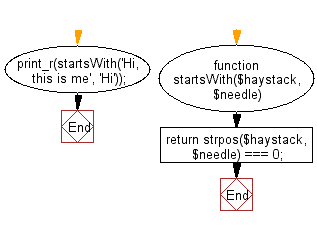﻿ PHP Exercise: Check if a given string starts with a given substring - w3resource

# PHP Exercises: Check if a given string starts with a given substring

## PHP: Exercise-95 with Solution

Write a PHP program to check if a given string starts with a given substring.

Sample Solution: -

PHP Code:

``````<?php
//Licence: https://bit.ly/2CFA5XY
function startsWith(\$haystack, \$needle)
{
return strpos(\$haystack, \$needle) === 0;
}
print_r(startsWith('Hi, this is me', 'Hi'));

?>
```
```

Sample Output:

```1
```

Flowchart:PHP Code Editor:

Have another way to solve this solution? Contribute your code (and comments) through Disqus.

What is the difficulty level of this exercise?

Test your Programming skills with w3resource's quiz.

﻿

## PHP: Tips of the Day

Returns all elements in an array except for the first one

Example:

```<?php
function tips_tail(\$items)
{
return count(\$items) > 1 ? array_slice(\$items, 1) : \$items;
}

print_r(tips_tail([1, 5, 7]));
?>
```

Output:

```Array
(
 => 5
 => 7
)
```